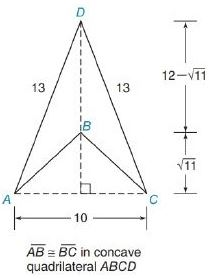Chapter 8.2, Problem 7E### Elementary Geometry for College St...

6th Edition
Daniel C. Alexander + 1 other
ISBN: 9781285195698

#### Solutions

Chapter
Section### Elementary Geometry for College St...

6th Edition
Daniel C. Alexander + 1 other
ISBN: 9781285195698
Textbook Problem
1 views

# In Exercises 1 to 8, find the perimeter of each polygon.To determine

To Find:

Explanation

Formula Used:

The perimeter of a polygon is the sum of the lengths of all sides of the polygon.

Pythagorean theorem for the right angle triangle ABC for the hypotenuse AC,

AC2=AB2+BC2

Calculation:

As the two sides of the triangles ADC and ABC are equal in length, both the provided triangles are isosceles.

Let the altitude of the triangle touches the base AC at E. Since the provided triangles are isosceles, its altitude divides the base AC into two equal parts with respect to E.

That is, AE=EC=102=5

### Still sussing out bartleby?

Check out a sample textbook solution.

See a sample solution

#### The Solution to Your Study Problems

Bartleby provides explanations to thousands of textbook problems written by our experts, many with advanced degrees!

Get Started

#### In Exercises 43-46, find f(a + h) f(a) for each function. Simplify yoiir answer. 44. f(x)=12x+3

Applied Calculus for the Managerial, Life, and Social Sciences: A Brief Approach

#### 0 1 π It does not exist.

Study Guide for Stewart's Multivariable Calculus, 8th

#### Distinguish between science and pseudoscience.

Research Methods for the Behavioral Sciences (MindTap Course List)